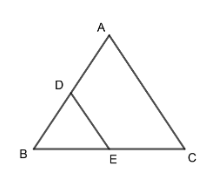Courses
Courses for Kids
Free study material
Free LIVE classes
MoreLIVE
Join Vedantu’s FREE Mastercalss

# In$\Delta ABC$, D is midpoint of AB, and E is midpoint of BC. Calculate: DE if AC = 6.4cm.Verified
361.5k+ views
Hint: Use the Mid Point theorem i.e. “The segment joining two sides of a triangle of their mid-points is always parallel to the third and it’s length is half of the third side.” You will get the final answer.To solve the above problem we will write the given data first, therefore,
In$\Delta ABC$,
D is the mid-point of side AB therefore,
Also E is the midpoint of side BC therefore we can write,
BE = CE
And AC = 6.4 cm. ………………………………. (1)
Now to find the value of ED we should know the concept given below,
Concept: (Mid-Point Theorem)
The segment joining two sides of a triangle of their mid-points is always parallel to the third and it’s length is half of the third side.
As we know that the segment ED joins at the midpoint of AB at D and also joins BC at its mid-point E. Therefore by using the above Mid Point Theorem we can say that the segment ED joins the two sides of $\Delta ABC$ at their midpoints and therefore segment ED is parallel and half of the third side AC.
$\therefore ED=\dfrac{1}{2}\left( AC \right)$
If we put the value of equation (1) in above equation we will get,
$\therefore ED=\dfrac{1}{2}\times \left( 6.4 \right)$
Therefore, ED = 3.2 cm.
As ED can be written as DE,
Therefore, DE = 3.2 cm
Therefore the value of segment DE is 3.2 cm.

Note: The mid-point theorem is only applied when the segment touches the midpoints of the two sides, so remember to check whether the segment is touching to the midpoints of two sides or not.
Last updated date: 25th Sep 2023
Total views: 361.5k
Views today: 10.61k